# Boson

In physics, a boson is an elementary particle with integral spin. According to the Pauli spin statistics postulate, systems of identical bosons are described by totally symmetric (under permutations of the bosons) wave functions.

A composite system of an even number of fermions may behave as a boson when the coupling between the constituting fermions is strong. For instance, atomic nuclei are composed of protons and neutrons; both types of nucleons are fermions. Atomic nuclei whose mass number A (the total number of nucleons) is even are bosons. Nuclei with odd A are fermions.

A thermodynamical system of N bosons satisfies Bose-Einstein statistics.

The boson is called after the Indian physicist Satyendra Nath Bose (1894–1974), who was the first to note that photons satisfying Planck's law for blackbody radiation obey a special kind of statistics, now called the Bose-Einstein statistics. (Photons have integral spin 1.)

• Note: this article lacks the point of view of particle physics.

## Mathematical description

Let an elementary boson have coordinates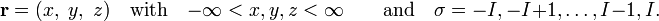$\mathbf{r} = (x, \;y,\; z) \quad \hbox{with}\quad -\infty < x,y,z < \infty\qquad\hbox{and}\quad \sigma = -I, -I+1, \ldots, I-1, I.$

The space coordinates x, y, and z are continuous and take on infinitely many values. The spin coordinate σ is discrete and can have 2I+1 different values. For bosons I is integral.

A one-boson wave function and an N-boson wave function are written as$\phi_a(\mathbf{r}_1,\sigma_1)\equiv \phi_a(1)\quad\hbox{and}\quad \Phi(1,2,\ldots,N) =\phi_1(1)\phi_2(2)\cdots\phi_N(N),$

where (k) stands for (rk, σk), k=1,...,N. Here Φ(1,2,...,N) is the simplest possible N-boson function (a product, which can be an exact solution of a many-boson Schrödinger equation only if the bosons do not interact). The N-boson function Φ, written here, has as a major defect: it does not satisfy Pauli's spin statistics postulate. This postulate states that the function must be symmetric under interchange of any two boson coordinates (under a "transposition").

### Examples

As a first example we take N = 2. The following function is not symmetric under interchange of 1 and 2 (given by the transposition operator P12), unless φa = φb,$\phi_a(1)\phi_b(2) \ne P_{12} \left[\phi_a(1)\phi_b(2)\right] = \phi_a(2)\phi_b(1)\quad\hbox{if}\quad \phi_a\ne\phi_b.$

The following (symmetrized) form is is invariant under P12, even when ab, and thus obeys the Pauli postulate: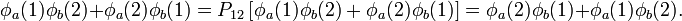$\phi_a(1)\phi_b(2) + \phi_a(2)\phi_b(1) = P_{12} \left[\phi_a(1)\phi_b(2) + \phi_a(2)\phi_b(1)\right]= \phi_a(2)\phi_b(1) + \phi_a(1)\phi_b(2) .$

For the second example we take N = 3 and write the following non-symmetric function with ab:$\phi_a(1)\phi_b(2)\phi_b(3) \ne \,P_{12} \left[\phi_a(1)\phi_b(2) \phi_b(3)\right] \equiv \phi_a(2)\phi_b(1)\phi_b(3)$

but$\phi_a(1)\phi_b(2)\phi_b(3)+\phi_a(2)\phi_b(1)\phi_b(3) = P_{12}\left[ \phi_a(1)\phi_b(2) \phi_b(3) +\phi_a(2)\phi_b(1)\phi_b(3)\right] = \phi_a(2)\phi_b(1)\phi_b(3)+\phi_a(1)\phi_b(2)\phi_b(3).$

It is easily verified that the latter function is not only symmetric under P12, but also under all 3! = 6 permutations of the three space-spin coordinates.

The last example shows that two bosons may occupy the same one-particle function (two bosons occupy φb). This is in contrast to fermions: as soon as two fermions occupy the same one-particle function, the total N fermion function vanishes.

## Composite systems of fermions

Let us consider a system consisting of N identical composite (non-elementary) subsystems, for instance a system consisting of N identical nuclei, where we recall that a nucleus is not elementary, but composed of neutrons and protons. One may determine whether the subsystems are bosons or fermions by considering the parity of the total wave function under the interchange of two subsystems. The subsystems are bosons if the wave function is symmetric (has even parity) under transpositions of the subsystems. The system consists of fermions if the parity of the wave function is odd (wave function obtains a minus sign) under transposition of the identical subsystems.

In order to show that composite subsystems consisting solely of fermions may behave as bosons, we recall that the Pauli statistics postulate requires fermionic wave functions to be antisymmetric (to have odd parity) under interchange of space-spin coordinates of any two identical fermions. Consider as an example a system consisting of N = 2 subsystems A and B, each itself consisting of two fermions. The fermions are labeled 1,..., 4. We assume that fermion 1 of A is identical to 3 of B and likewise that 2 and 4 are identical. A system wave function is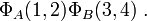$\Phi_A(1,2)\Phi_B(3,4) \; .$

If both fermions of A (1 and 2) and of B (3 and 4) are identical one may expect the intra-subsystem transpositions 1 ↔ 2 and 3 ↔ 4 to enter the discussion as well. However, when there is strong coupling within the subsystems (for instance by nuclear forces), permutations that effectively interchange 1 and 2 and/or 3 and 4 are not feasible and do not have to be considered. The only relevant permutation is then the transposition of A and B,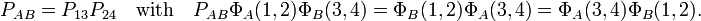$P_{AB} = P_{13}P_{24} \quad\hbox{with}\quad P_{AB} \Phi_A(1,2)\Phi_B(3,4) = \Phi_B(1,2)\Phi_A(3,4) =\Phi_A(3,4)\Phi_B(1,2).$

Since the interchange of A and B consists of two transpositions of fermions, it gives the sign (−1)×(−1) = 1 and hence the identical systems A and B are bosons (i.e., their wave function is symmetric under transposition). The wave function must be symmetrized,$\Phi_A(1,2)\Phi_B(3,4) + \Phi_B(1,2)\Phi_A(3,4)\; \quad\hbox{for} \quad\Phi_A \ne \Phi_B.$

in order to obey the Pauli postulate.

In this way one can explain why an ideal gas, which by definition consists of non-interacting particles, is sometimes bosonic and sometimes fermionic. For instance, an ideal gas of H-atoms is bosonic (an H-atom consists of two fermions: one electron and one proton), while an ideal gas consisting of D (= 2H) atoms is fermionic. Deuterium consists of three fermions: a proton, a neutron, and an electron. Simultaneous transpositions within the three pairs of fermions, which is equivalent to the interchange of two D-atoms, gives (−1)3 = −1.

Finally, it must be noted that for non-ideal gases the conclusions can be different. If, for example, we turn on the chemical interaction between the D-atoms in the fermionic ideal gas of D-atoms, D2 molecules will be formed. A D2 molecule consists of an even number of fermions and hence a gas of D2 molecules is a boson system.

## Complete sets of orbital products

Consider a linear space VM spanned by the M boson spin-orbitals φ1, φ2, ..., φM. We assume these orbitals to be orthonormal. Consider the tensor power$V_M \otimes^N \equiv \underbrace{V_M\otimes V_M\otimes \cdots\otimes V_M}_{N \mathrm{factors}}$

The tensor power is of dimension MN and the symmetrized states are in the subspace$W\equiv\mathcal{S}\big(V_M \otimes^N\big)$, which is of dimension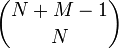$\binom{N+M-1}{N}$. An unnormalized basis (maximum linearly independent set) for W is the following set$\big\{ \mathcal{S}\big(\phi_{i_1}\otimes \phi_{i_2}\otimes \cdots\otimes \phi_{i_N}\big) \;;\; i_1 \le i_2 \le \cdots \le i_N \big\} .$

Example. M = 3 and N = 3, the number of symmetrized states is$\binom{5}{3} = 10$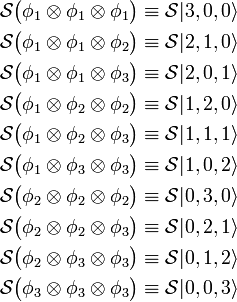\begin{align} \mathcal{S}\big(\phi_{1}\otimes \phi_{1}\otimes \phi_{1}\big) &\equiv \mathcal{S}| 3, 0, 0\rangle \\ \mathcal{S}\big(\phi_{1}\otimes \phi_{1}\otimes \phi_{2}\big) &\equiv \mathcal{S}| 2, 1, 0\rangle \\ \mathcal{S}\big(\phi_{1}\otimes \phi_{1}\otimes \phi_{3}\big) &\equiv \mathcal{S}| 2, 0, 1\rangle \\ \mathcal{S}\big(\phi_{1}\otimes \phi_{2}\otimes \phi_{2}\big) &\equiv \mathcal{S}| 1, 2, 0\rangle \\ \mathcal{S}\big(\phi_{1}\otimes \phi_{2}\otimes \phi_{3}\big) &\equiv \mathcal{S}| 1, 1, 1\rangle \\ \mathcal{S}\big(\phi_{1}\otimes \phi_{3}\otimes \phi_{3}\big) &\equiv \mathcal{S}| 1, 0, 2\rangle \\ \mathcal{S}\big(\phi_{2}\otimes \phi_{2}\otimes \phi_{2}\big) &\equiv \mathcal{S}| 0, 3, 0\rangle \\ \mathcal{S}\big(\phi_{2}\otimes \phi_{2}\otimes \phi_{3}\big) &\equiv \mathcal{S}| 0, 2, 1\rangle \\ \mathcal{S}\big(\phi_{2}\otimes \phi_{3}\otimes \phi_{3}\big) &\equiv \mathcal{S}| 0, 1, 2\rangle \\ \mathcal{S}\big(\phi_{3}\otimes \phi_{3}\otimes \phi_{3}\big) &\equiv \mathcal{S}| 0, 0, 3\rangle \\ \end{align}

Here we exemplified the "occupation number notation" in which an arbitrary element is written as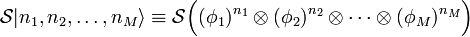$\mathcal{S}| n_1, n_2, \ldots, n_M\rangle \equiv \mathcal{S}\Big( (\phi_1)^{n_1}\otimes(\phi_2)^{n_2}\otimes\cdots\otimes(\phi_M)^{n_M}\Big)$

where occupation numbers nk, k=1, ..., M, may be zero. A complete basis for the symmetrized space W is labeled by all possible decompositions of N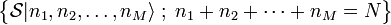$\big\{ \mathcal{S}| n_1, n_2, \ldots, n_M\rangle\; ; \; n_1+n_2+ \cdots + n_M = N \big\}$

The symmetrized products are orthogonal but not normalized. In this article it is shown that their normalization coefficient is the square root of a multinomial coefficient: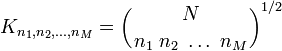$K_{n_1,n_2,\ldots,n_M} = \left({ N \atop n_1\;n_2\;\ldots\;n_M}\right)^{1/2}$

In conclusion, the following set of symmetrized states forms an orthonormal basis for W: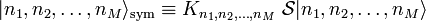$| n_1, n_2, \ldots, n_M\rangle_{\mathrm{sym}} \equiv K_{n_1,n_2,\ldots,n_M}\;\mathcal{S}| n_1, n_2, \ldots, n_M\rangle$

where the M occupation numbers are natural numbers (positive or zero) with the constraint that they sum up to N.

### Application

The trace of a linear operator A can be expressed in terms of an orthonormal basis. The canonical partition function Q is the trace of exp(−βH), where H is an energy operator and β ≡ 1/(kT). (Here k is Boltzmann's constant and T is the absolute temperature.)

As an example of a statistical physics trace calculation we consider a Hamiltonian of a system of N non-interacting bosons:$H = h(1) + h(2) + \cdots + h(N)$

and assume that the one-particle space VM is spanned by eigenstates:$h \phi_{i} = \epsilon_i \phi_i \Longrightarrow e^{-\beta h} \phi_i = \lambda_i \phi_i \quad\hbox{with}\quad \lambda_i \equiv e^{-\beta \epsilon_i}.$

The operator H commutes with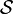$\mathcal{S}$ so that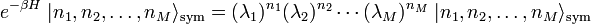$e^{-\beta H}\; | n_1, n_2, \ldots, n_M\rangle_{\mathrm{sym}} = (\lambda_1)^{n_1}(\lambda_2)^{n_2} \cdots (\lambda_M)^{n_M} \; | n_1, n_2, \ldots, n_M\rangle_{\mathrm{sym}}$

From this follows$Q\equiv\mathrm{Tr} e^{-\beta H} = \sum_{n_1, n_2, \dots, n_M \atop n_1+n_2+\cdots+n_M = N} (\lambda_1)^{n_1}(\lambda_2)^{n_2} \cdots (\lambda_M)^{n_M}$

because the symmetrized states are normalized. Compare this with the multinomial expansion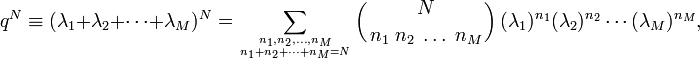$q^N \equiv (\lambda_1 + \lambda_2 + \cdots + \lambda_M)^{N} = \sum_{n_1, n_2, \dots, n_M \atop n_1+n_2+\cdots+n_M = N} \left({ N \atop n_1\;n_2\;\ldots\;n_M}\right) (\lambda_1)^{n_1}(\lambda_2)^{n_2} \cdots (\lambda_M)^{n_M},$

where q is the one-boson partition function. In statistical physics M is usually infinite, the space VM is a one-boson Hilbert space. When the temperature T is fairly high, not many states are occupied more than once. For most terms in the sum we have occupation numbers 1 or 0. If we make the approximation that this is true for all terms, the multinomial coefficient becomes equal to N! and we obtain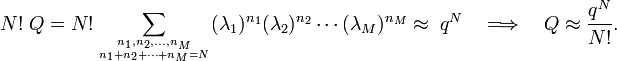$N!\;Q = N! \sum_{n_1, n_2, \dots, n_M \atop n_1+n_2+\cdots+n_M = N} (\lambda_1)^{n_1}(\lambda_2)^{n_2} \cdots (\lambda_M)^{n_M} \approx \; q^N \quad\Longrightarrow\quad Q \approx \frac{q^N}{N!}.$

This approximation is known as Boltzmann statistics.